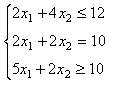# Simplex method calculator - : Solve the Linear Programming Problems Easily

## Simplex method calculator

Type your linear programming problem below. (Press "Example" to see how to set it up.)

Mode:

Rounding (significant digits):

Solution:

The tableaus (steps) will appear here.

Check out the best and amazing linear programming calculator tool with us. This calculator is an amazing tool that can help you in solving the linear programming equations with ease. With the motive to help you in making your calculations simple and interesting, we have designed this tool for you. All you need to do is to input your function, and it will show you the result easily within seconds. For solving the linear programming problems, the simplex method has been used.

In order to help you in understanding the simplex method calculator with steps, we have taken a linear programming problem that is minimizing the cost according to the constraints.
Cost: C= 5x1 + 3x2
The constraints are:### Step 1

First of all, the initial tableau will be set up. First of all, you need to decide what your objective is to minimize or maximize the cost. Additionally, you need to decide how many variables are there in the constraints and what the type of the constant is. On the basis of this information, that tableau will be created of the right size. You need to complete it by entering the names of the variables and the coefficients that are appeared in the constants and the objective function as well.

### Step 2

Applying the simplex method First of all, you need to choose the column and leave the row. The same procedure will be followed until the solution is availed. On the status bar, you will get to know about the continuation of the steps. Once the process is completed, you will get the final solution to your problem.

## Simplex algorithm

It is one of the popular methods that are used to avail of the numerical solution of linear programming problems. The best part about this calculator is at it easily solving the problems accurately within small steps. If you want to optimize your practice, then you must use the linear programming simplex method calculator.

### Linear programming

The simplex method is one of the popular solution methods that are used in solving the problems related to linear programming. The two variables and constraints are involved in this method. In this, basic variables are the solutions given for the constraint equation having non-zero variables. In order to get the optimal value of the objective function, this systematic method is used.

### Simplex algorithm calculator

The online simplex method calculator or simplex solver, plays an amazing role in solving the linear programming problems with ease. The best part about this calculator is that it can also generate the examples so that you can understand the method.

### Two-Phase Simplex Method Calculator

The calculator given here can easily solve the problems related to the simplex method, two-phase method, and the graphical method as well. In this calculator, you can enter a limitless number of variables or constants.
The best part about this maximization calculator is that you do not need to have any language to state the problem specifically. The user interface of this tool is so easy that any user without having any technical knowledge can use it.

## Points to remember while using the calculator:

• Do not use commas in large numbers while using the simplex tableau calculator.
• On the right-hand side of each constant do not enter any e negative number.
• In the decimal mode, all the results will be displayed in decimals.
• The fraction mode helps in converting all the decimals to the fractions.
• The interior mode helps in eliminating the decimals and fractions from the tables.

## Dual Simplex Method Calculator

The dual simplex method maximization calculator plays an important role in transforming an initial tableau into a final tableau. The Final Tableau always contains the primal as well as the dual problems related solutions. Each stage of the algorithm plays amazingly in generating an intermediate tableau as the algorithm scrabbles towards the final result.
Finally, these are all the essential details regarding the simplex calculator. You can easily use this calculator and make your simple linear programming equation problems easy and simple as well.